# Thoughts on Perfect Impedance Matching of a Yagi

## By: John Magliacane, KD2BD

#### A Follow-up To "Perfect Impedance Matching Of A Yagi" by Dick Knadle, K2RIW, 2005, 2006

A half-wave dipole illuminating a parasitic array will often require a "step-up" in feedpoint impedance to properly match the surge impedance of popular transmission lines. Extending the physical length of a monopole or dipole causes a slow rise in radiation resistance. Contracting the length has the opposite effect. This phenomenon is illustrated in the Brown-Woodward curves published in Chapter 4 of the Antenna Engineering Handbook: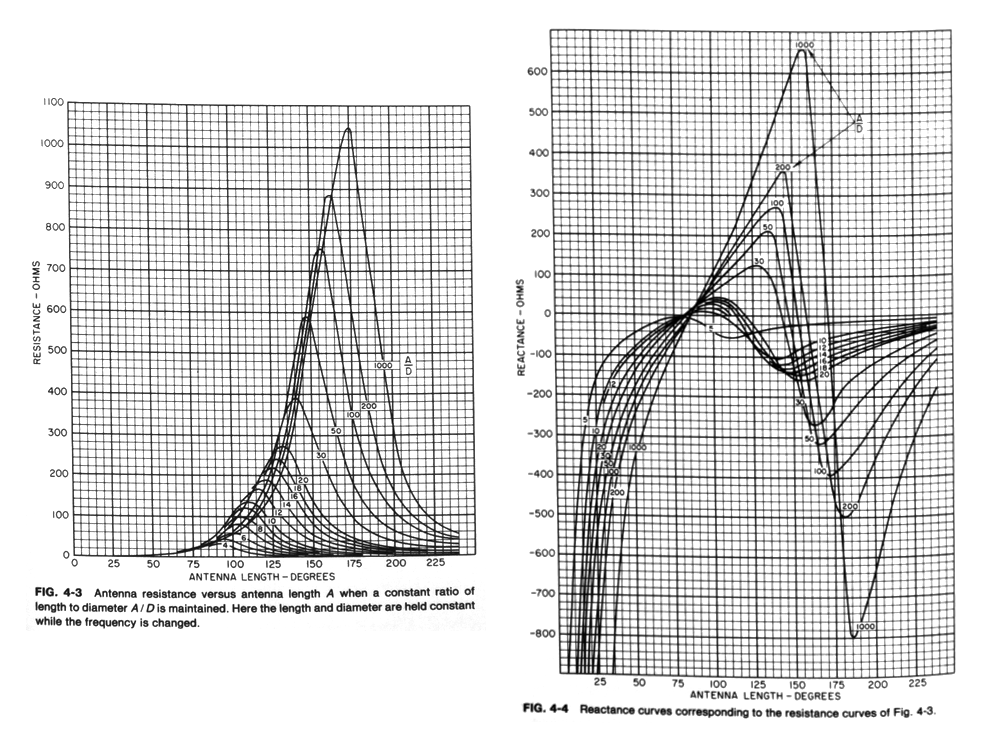This often ignored property of monopole and dipole antennas makes it possible to achieve a wide spectrum of feedpoint impedances suitable for virtually any application.

The only consequence of modifying the radiator's physical length beyond the point where natural resonance occurs is that the feedpoint impedance becomes highly reactive. In fact, the reactance varies more rapidly in magnitude than does radiation resistance as the length of the radiator is moved away from resonance.

The feedpoint impedance's reactive component is easily cancelled by placing conjugate reactances in series with the feedpoint of the radiator. When the added reactance is made equal in magnitude and opposite in phase of that presented by the radiator, a pure resistive impedance equal to that predicted by the Brown-Woodward curves is realized.

A shunt reactance can be placed in parallel with the feedpoint to cancel the reactive component of the driven element's impedance as well. However, what happens in the process is rather complex, and is not as trivial as simply making the shunt reactance equal and opposite to that of the driven element's reactance. Instead, a parallel resonant RLC circuit is formed, and the radiation resistance seen at the feedpoint is stepped up in the process to a level significantly higher than what the Brown-Woodward curves alone predict.

Provided a step-up in feedpoint impedance is desired, a wide range of impedances can be obtained simply by selecting the radiator's physical length and shunt reactance appropriately. In fact, it almost doesn't matter whether the driven element is adjusted to a physical length above or below resonance. There's likely an impedance matching solution for either situation.

## Shunt Capacitance Impedance Matching Analysis

When a driven element exhibiting inductive reactance is brought to resonance by introducing a capacitive reactance in parallel with the element's feedpoint, the following equivalent RLC circuit is developed:The shunt capacitive reactance, XC, required to resonate a complex load exhibiting a pure resistance, R, in series with a distributed inductive reactance, XL, can be computed as follows: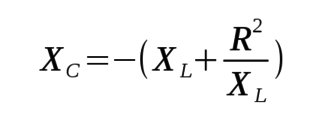After applying the shunt capacitive reactance, XC, across the driven element to bring the system into resonance, the resulting feedpoint resistance, Rfeedpoint, can be computed as follows: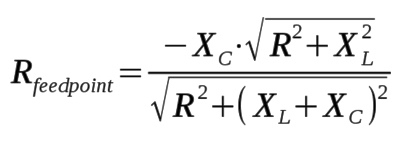Note that all resistances and reactances are expressed in ohms, with XC expressed as negative in magnitude.

## Shunt Inductance Impedance Matching Analysis

A very similar impedance matching function, including a step-up in feedpoint impedance, can be realized if a shunt inductance is connected across the feedpoint of a driven element that is shorter than physical half-wave resonance. Here is the equivalent RLC circuit: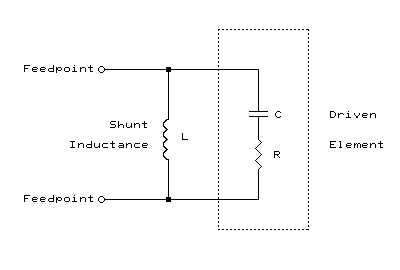The shunt inductive reactance, XL, required to resonate a complex load exhibiting a pure resistance, R, in series with capacitive reactance, XC, can be computed as follows: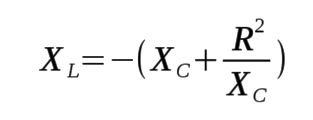After applying the shunt inductance, XL, across the driven element to bring the system into resonance, the resulting feedpoint resistance, Rfeedpoint, can be computed as follows: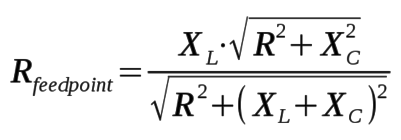In other words, much like the shunt capacitance impedance matching technique, a step-up in feedpoint impedance is realized by applying a shunt inductance across a capacitive load, despite the reduction in radiation resistance caused by decreasing the length of the radiating element to produce the needed capacitive reactance.

Shunt inductance impedance matching is often employed in HF mobile antenna installations when the radiating element is less than a quarter-wavelength in height and an impedance step-up to match a 50 ohm transmission line is desired. A "loading coil" of suitable inductance is connected in series between the bottom of the radiating element and ground. If the element length and the inductance of the coil are properly chosen, not only is resonance established, but a 50 ohm feedpoint impedance across the coil is also achieved.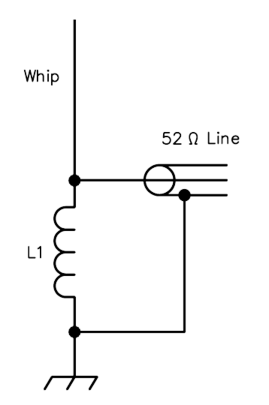Shunt Inductance Impedance Matching for a Less Than One Quarter Wavelength Monopole

Another example of where shunt inductance impedance matching is employed is in "Hairpin" Impedance Matching Networks.

## Hairpin Impedance Matching

A "hairpin" is a shunt inductance placed across the feedpoint of a capacitively reactive load to raise the resistive component of its impedance. It is typically fabricated from parallel lengths of wire forming a balanced transmission line that is less than 45 degrees in length and shorted on the far end, making it purely inductive.

For many years, the ARRL Handbook published a design for a 5-element 50 MHz yagi that employed a "hairpin" across the antenna's dipole driven element to raise its impedance to 200 ohms. Connection to a 50-ohm unbalanced feed-line was made through a 4:1 half-wave coaxial balun. The description of the antenna cautions the reader that the driven element is the shortest element of the entire array. The article states, "While this may seem a bit unusual, it is necessary with the hairpin matching system".

## Relationship To L-Network Impedance Matching

The reason these impedance matching techniques produce an impedance step-up in either configuration is because they are really L-networks with their high-impedance, shunt reactance side positioned at the feedpoint, and their low-impedance, series reactance side connected in series with the driven element's radiation resistance:Shunt Capacitance Equivalent Circuit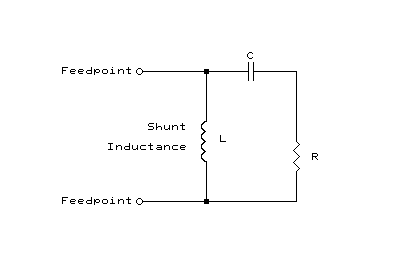Shunt Inductance Equivalent Circuit

Another interesting relationship occurs with these networks. When the network is properly adjusted to the point of resonance, the ratio of radiation resistance to series reactance is always equal to the ratio of shunt reactance to feedpoint resistance. Knowing this relationship makes the calculation of feedpoint resistance significantly easier than previously described once the shunt reactance is first determined.

## Some Yagi Impedance Matching Examples

Using YAGIMAX 3.11 software, the feedpoint impedance of 18 element 432 MHz DL6WU Yagi was estimated to be 34.23+j0.24 ohms using a 33.1978 cm long driven element (dipole) at 432.200 MHz. Such a termination impedance would produce a VSWR of appoximately 1.52:1 on a 52 ohm transmission line.

If the driven element is lengthed to 34.450 cm (below resonance), the feedpoint impedance rises to 42.43+j20.06 ohms. Using the equations illustrated above, when a capactive reactance of 109.8 ohms (3.35 pF) is placed across the feedpoint of the driven element, a 51.9 ohm purely resistive feedpoint impedance is obtained.

Going in the opposite direction, if the driven element is shortened to 31.6 cm (above resonance), a feedpoint impedance of 26.07-j25.95 ohms is obtained. Using the equations illustrated above, when an inductive reactance of 52.14 ohms (19.2 nH) is placed across the feedpoint of the driven element, a 51.9 ohm purely resistive feedpoint impedance is again obtained.

A balun is required to symmetrically feed a balanced driven element with an unbalanced coaxial transmission line. 4:1 half-wave coaxial baluns are often used, and require that the feedpoint of the Yagi be 200 ohms in order to yield a 50 ohm output impedance.

If the driven element is lengthed to 39.57 cm, a feedpoint impedance of 102.03+j100.11 ohms is obtained. A shunt capactive reactance of 204.1 ohms (1.8 pF) across the feedpoint yields a purely resistive impedance of 200.2 ohms. Note that under this tuning condition, the driven element is physically 13% longer than the reflector, but is the proper electrical length for the Yagi to function correctly.

Going in the opposite direction, if the driven element is shortened to 29.665 cm, the feedpoint impedance becomes 18.71-j58.30 ohms. A shunt inductive reactance of 64.3 ohms (23.67 nH) yields a resistive feedpoint impedance of 200.3 ohms. Under this tuning condition, the driven element is shorter than the first four directors, but as before, is the proper electrical length to function correctly.

## Shunt Capacitance Impedance Matching Network Calculator

The following Javascript calculator will determine a dipole's radiation resistance once the resistive and inductive reactive components of the dipole's feedpoint impedance are known, and the indicated shunt capacitance is applied across the feedpoint terminals:

Resistance (R) of Driven Element: Reactance (XL) of Driven Element: Frequency (MHz):

------------------------------------------------------------------ ------------------------------------------------------------------

After applying a shunt capacitive reactance (XC) of ohms, your feedpoint resistance becomes ohms.
At your frequency of operation, this amount of XC equates to pF of shunt capacitance.

## Shunt Inductance Impedance Matching Network Calculator

The following Javascript calculator will determine a dipole's radiation resistance once the resistive and capacitive reactive components of the dipole's feedpoint impedance are known, and the indicated shunt inductance is applied across the feedpoint terminals:

Resistance (R) of Driven Element: Reactance (XC) of Driven Element: Frequency (MHz):

------------------------------------------------------------------ ------------------------------------------------------------------

After applying a shunt inductive reactance (XL) of ohms, your feedpoint resistance becomes ohms.
At your frequency of operation, this amount of XL equates to nH of shunt inductance.

## Conclusion

Whether the feedpoint impedance of a Yagi's driven element is raised using a shunt capacitance and a slightly longer-than-naturally resonant driven element length, or a shunt inductance and slightly shorter-than-naturally resonant driven element length may be academic. In either case, the step-up in feedpoint impedance occurs that is due primarily to the impedance transformation nature of the network itself in the shunt capacitance scenario, and entirely due to the impedance transformation nature of the network in the shunt inductance impedance matching scenario.

The shunt inductance method has the advantage of providing a DC (static discharge) path across the radiator, while the shunt capacitance method utilizes a radiator having a higher radiation resistance, which results in a matching network having a lower 'Q' and a wider operating bandwidth.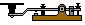John Magliacane Amateur Radio Operator: KD2BD